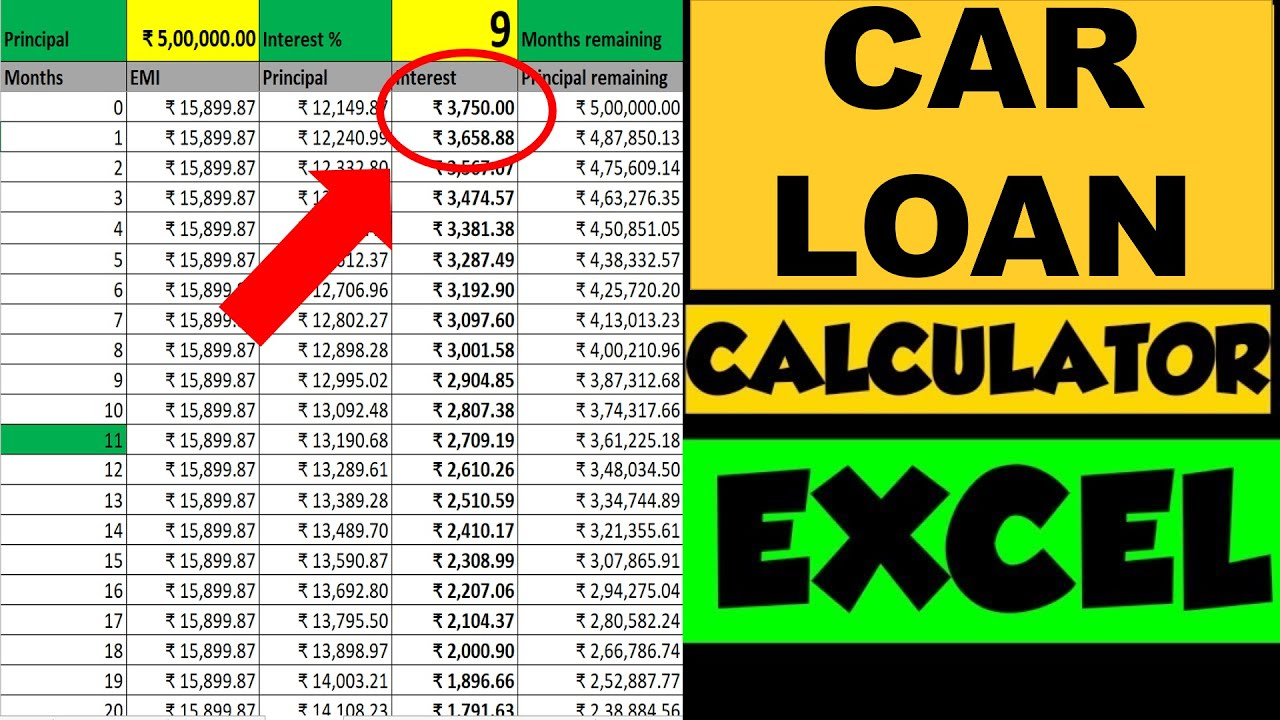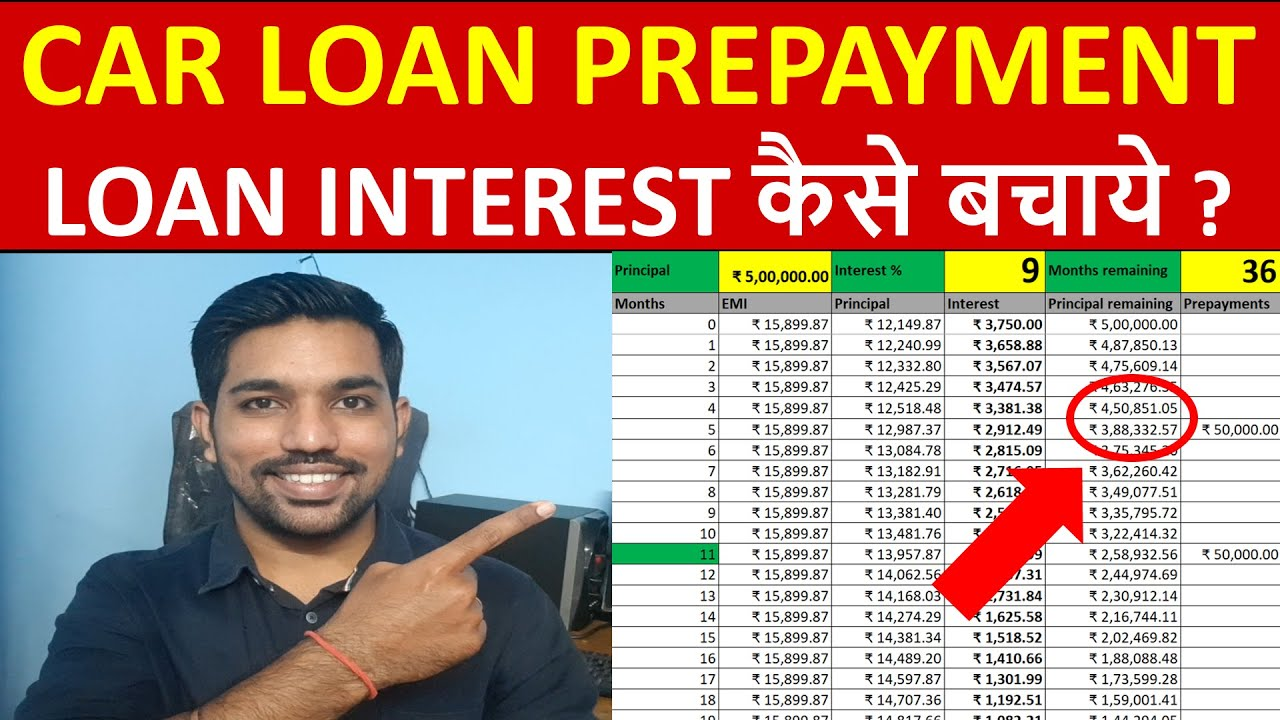# SBI Car Loan Calculator

Share this Free SBI Car Loan EMI Calculator with your friends and family members and help them in SBI Car Loan EMI Calculation!

Watch below video to understand SBI Car Loan EMI calculation in excel:

## SBI Car Loan EMI Calculation – VideoWatch more Excel Videos Here

## THINGS TO KNOW ABOUT SBI CAR LOAN EMI

• There are 2 parts of your EMI: Principal amount Interest Amount
• Interest Amount is high initially during your Car Loan tenure
• Principal amount is less initially during your Car Loan tenure
• High interest amount is already taken at the initial Car loan period from your EMIs
• You should pre pay most of your principal amount if you want to decrease maximum interest amount in your Car Loan
• You can easily calculate your Car loan EMI using excel calculator as we can see in above video
• Pre pay your Car loan principal amount initially as much as you can to decrease interest amount that you have to pay throughout your loan tenure
• Increase in car loan tenure

## How is SBI Car Loan EMI Calculated Monthly?

It is very easy to calculate EMI of car loan using above calculator. Here are the steps to calculate car loan EMI:

1. Provide Loan Amount you will be taking from bank or financial institution
2. Enter yearly interest rate of car loan
3. Enter tenure in months
4. That’s it. These 3 numbers will give you the appropriate EMI for your car loan

Below is the screenshot of the calculation considering, Rs. 5 Lakh car loan amount, 9% interest rate and 36 months tenure:

As seen above, Rs. 15,900 is the car loan EMI amount (every month installment), Rs. 72,395 is the total interest you’ll pay throughout the loan tenure and Rs. 5,72,395 is the total amount you will be paying back.

It is important to note that your EMI amount consists of 2 components: Principal Amount and Interest Amount.

Principal Amount is low initially and increases with time as you pay your car loan EMI.

Interest Amount is high initially and decreases with time as you pay your car loan EMI.

This shows that maximum possible amount of interest from your car loan is taken from you during the initial stages (or months) of your car loan EMI repayment.

You can use below methods to save car loan interest:

### How to Save Car Loan Interest?

Below are some ways to save car loan interest:

• Check for low interest rate car loan
• Keep the tenure low. Higher the tenure, longer you will keep paying EMI thus increasing the overall interest amount. So keep tenure low
• Make Car Loan Prepayments whenever possible. Prepayment is a method to pay additional amount to reduce your principal outstanding balance on your loan.
• Keep the loan amount as low as possible by doing maximum down payment for your car.
• Check interest rates in banks and compare with showrooms

Watch below video on How Car Loan Prepayment can help you save interest with help of examples in excel:

### Car Loan Prepayment in Excel – VideoWatch more Excel Videos Here

You may also like Home Loan Prepayment method if you have Home Loan EMI as well.

## What is Car Loan Repayment Table?

So far we have understood how to calculate car loan EMI using calculator. Let us now go one step further and see how car loan EMI will be divided in 2 components using car loan repayment table.

This car loan repayment table is the table that shows you every month EMI amount (that is kept unchanged) and details of principal and interest amounts from this EMI, which changes every month.

We will take same example as above.

Car Loan Amount = Rs. 5,00,000
Interest Rate = 9% per annum
Tenure = 36 Months

Below is the car loan repayment table:

As seen in above car loan repayment table (only first 10 months displayed), EMI of Rs. 15,900 in first month is divided into 2 parts – Rs. 12,150 (Principal Amount) and Rs. 3,750 (Interest Amount).

Further, as you will notice, the principal amount increases in 2nd month, but the interest amount decreases in same 2nd month. This continues till the end of loan tenure.

You can check calculations for entire car loan tenure by using this Car Loan Excel calculator.

## Benefits of using SBI Car Loan EMI Calculator

There are multiple benefits of using car loan calculator:

• You will know correct car loan EMI. No showroom can provide you incorrect quotes and misguide you
• This calculator gives you the idea of EMI to check if you can afford
• You can also adjust the tenure in months and get the EMI in affordable range
• It gives you the EMI amount for various interest rates that you can compare between banks such as SBI, ICICI, Axis, HDFC, etc.

More Recommended Books Here

## What is the EMI for 10 Lakh Car?

Considering that entire 10 Lakh will be taken as loan and Using the calculator and below numbers for your car loan:

Car Loan Amount = Rs. 10 Lakh
Interest rate = 9%
Tenure = 5 Years

We get the car loan EMI as Rs. 20,758

Below is the screenshot of calculation:

If you can make down payment of Rs. 2 Lakh and take loan of 8 Lakh, below is the calculation:

Car Loan Amount = Rs. 8 Lakh
Interest rate = 9%
Tenure = 5 Years

We get the car loan EMI as Rs. 16,607

Below is the screenshot of calculation:

So as seen above, with reduction of loan amount, EMI is also decreased. That’s the reason we should take loan for amount that is required. Not less not more.

## Is 7% good for Car Loan?

7% interest rate is really good for car loan. Currently the home loan interest rates starts from 7.5% (as of writing this article).

Car loan interest rates will be always higher than home loans. So anything below 10% should be good interest rate for car loan, provided you try to minimize the loan amount and keep tenure low.

## What is Car Loan Prepayment to Save Loan Interest

Car Loan Prepayment is the method to save car loan interest.

Prepayment is a method to pay additional amount and reduce your outstanding principal amount. Your remaining principal amount is the important factor in car loan, as it calculates the monthly interest amount as part of your EMI.

So you should try to reduce this remaining principal balance to save car loan interest amounts.

For example, in above example of car loan, you pay total of Rs. 72,395 as the interest amount in 36 months. But just be paying additional Rs. 50,000 in 5th month, you can save Rs. 12,804 of loan interest amount.

Updated Interest amount will be Rs. 59,591.

Below is the screenshot of calculations:

Watch this video to know more about car loan prepayment in excel with examples.

## What is the interest rate of SBI car loan?

Car Loan Interest rates in SBI can range anywhere between 8% to 15% per annum. Lower the interest rate, low interest amount you will be paying.

Further, loan interest rates for electric vehicles (EV) are lower compared to normal vehicles that run on fuel. This is to promote buying of electric vehicles using SBI car loan.

## How much car loan can I get on 40000 salary?

On your salary of Rs. 40000, you should try to keep the loan EMI affordable for you every month. The ideal number is to have no more than 20% of your monthly income as car loan EMI.

So you should try to get your car loan EMI within Rs. 10,000, and for 5 years tenure, you should be able to get maximum of Rs. 5 Lakh car loan on your salary of Rs. 40,000.

We have considered 9% interest rate and 60 months tenure. If interest rate increases or tenure decreases, than the loan amount you are eligible for might also decrease.

## At what salary person should buy car?

It doesn’t matter at what age you are (provided you have car license to drive). What you should focus on is the car loan EMI.

• Do not have more than 50% of your income going in your car loan EMI or any other EMI
• Try to take minimum loan amount so that you can close loan early
• Remember that car is a liability, so allocate funds accordingly if you are planning to buy one
• If you really need a car and can afford at least Rs. 10,000 to Rs. 15,000 of EMI to be paid for next 5 years, you can buy a car within Rs. 8 Lakh of car amount.
• Regarding age – you can be 18 years old or 55 years old and can follow above points, there is no such specific criteria or age to be checked to buy a car

## Conclusion

While you are excited to buy your new car or already bought one on loan, it is important to calculate correct car loan EMI.

By using above car loan EMI calculator, you can check the EMI, total interest amount and total amount you’ll be paying in your loan tenure.

While doing so, also remember to make car loan prepayments in order to save loan interest which you can use to invest in mutual funds or any fixed income assets like Fixed Deposits, Recurring Deposits, etc.

## You can Donate Too!

Found this Helpful? DONATE any amount to see more useful Content. Scan below QR code using any UPI App!

UPI ID: abhilashgupta8149-1@okhdfcbank

Verify that you are “Paying Abhilash Gupta” before making the transaction so that it reaches me. It makes my Day 🙂

Thank you for Donating. Stay Tuned!

## Income Tax Calculator App – FinCalC

For Income Tax Calculation on your mobile device, you can Download my Android App “FinCalC” which I have developed for you to make your income tax calculation easy.

### What you can do with this mobile App?

• Calculate Income Tax for new FY 2023-24 and previous FY 2022-23
• Enter estimated Investments to check income tax with Old and New Tax Regime
• Save income tax details and track regularly
• Know how much to invest more to save income tax
• More calculators including PPF, SIP returns, Savings account interest and lot more